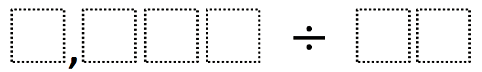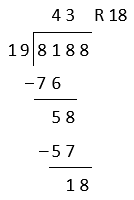# Multiplication and Division of Whole Numbers

## Objective

Divide multi-digit numbers by one- and two-digit divisors and assess the reasonableness of the quotient.

## Common Core Standards

### Core Standards

?

• 5.NBT.B.6 — Find whole-number quotients of whole numbers with up to four-digit dividends and two-digit divisors, using strategies based on place value, the properties of operations, and/or the relationship between multiplication and division. Illustrate and explain the calculation by using equations, rectangular arrays, and/or area models.

?

• 4.NBT.B.4

• 4.NBT.B.6

• 5.NBT.A.1

• 5.NBT.A.2

## Criteria for Success

?

1. Estimate partial quotients of up to four-digit dividends and up to two-digit divisors with up to three-digit quotients.
2. Use the estimate to approximate each value in the quotient, adjusting when the estimate is either too high or too low.
3. Divide up to four-digit dividends by up to two-digit divisors with up to three-digit quotients and remainders in the ones place.
4. Check that the solution to a division problem is correct by using inverse operations, multiplying the quotient by the divisor and adding the remainder, seeing if it is equivalent to the dividend.
5. Assess the reasonableness of an answer by rounding and/or using the relationship between multiplication and division to check answers (MP.1).
6. Solve word problems involving division, including those that require interpretation of the remainder (MP.1, MP.4).

#### Fishtank Plus

• Problem Set
• Student Handout Editor
• Vocabulary Package

?

### Problem 1

Make the largest quotient by filling in the boxes using the whole numbers ${1-9}$ no more than one time each.#### References

Open Middle Dividing Two-Digit Numbers (Elementary)

Dividing Two-Digit Numbers (Elementary) by is made available on Open Middle under the CC BY-NC-SA 4.0 license. Accessed Dec. 5, 2017, 4:13 p.m..

Modified by The Match Foundation, Inc.

### Problem 2

a.      ${8,801\div33}$

b.      ${6,132\div15}$

c.     ${7,464 \div 92}$

## Discussion of Problem Set

?

• Look at #1b. What did you do when you got to the tens place?
• Look at #1c. What did you do when you got to the tens place?
• What made #1h easier than #1g or #1j?
• Look at #1. Were there any problems where your estimate was incorrect and you had to adjust it?
• I think the principal now needs to buy 54 cartons of eggs in #3b. I saw a student write that the principal now needs to buy 53 cartons of eggs. Who do you agree with? Why?

?

### Problem 1

For parts (a) and (b) below,

• Solve. Show or explain your work.
1.   $1,534\div6$
2.   $344\div18$

### Problem 2

Henry says the solution to ${8,{18}8\div19 }$ is ${43}$ with a remainder of ${18}$. His work is shown below.• Use estimation to explain why Henry’s answer is not reasonable.
• What error do you think Henry made? Why do you think he made that error?
• Find the correct quotient of ${8,{18}8\div19 }$

?